Mechanics

# Free fall and vertical launch

Freefall and vertical launch are one- dimensional , uniformly accelerated motion . These movements occur, respectively, when a body is released from rest from a certain height or when launched in a vertical direction . Since the acceleration of gravity is constant, if we disregard the action of dissipative forces, the ascent and descent times in these types of movements will always be the same.

## Free fall

In practice, the ideal free-fall motion is quite close to that in which an object is dropped at a small height above the ground. However, strictly speaking, this motion only happens when some object is dropped into a vacuum . According to the free fall equations of motion, the time of fall does not depend on the mass of the objects, but on the acceleration of gravity and the height at which that object is dropped.

Free fall is a vertical movement that occurs with constant acceleration, so that the falling speed of the body increases with each second in relation to the center of the Earth, according to the local acceleration of gravity.

When released in a vacuum , bodies of different masses will reach the ground at the same time. The fact that a feather does not reach the ground at the same time as a bowling ball, when dropped to the Earth’s surface, is associated with air friction , which is almost negligible for heavy , aerodynamic objects such as the bowling ball.

## free fall formulas

Here are the main formulas that are used for free fall calculations:

• ### Speed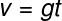v – speed

g – gravity

t – time

• ### Height in relation to time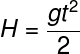H – height

• ### Speed ​​in relation to height

Finally, the following equation relates the speed of fall to the height without needing the time variable. This equation is obtained from the Torricelli equation , used for the cases of uniformly varied motion as well as free fall: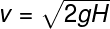## vertical launch

Unlike free fall, in vertical launch, the initial velocity is non-zero , that is, the object starts moving by being pushed up or down . Since the acceleration of gravity is constant when thrown upwards, the time it takes for the thrown object to reach maximum height is equal to the downward motion.

## Vertical launch formulas

• ### Hourly equation of speed

The simplest vertical launch formula is the hourly velocity equation In this formula, there is a negative sign , as we are considering an object being thrown from the bottom up , that is, the acceleration of gravity slows it down .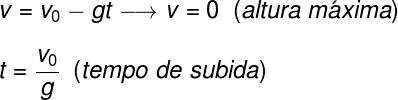v – final speed

v0 – initial speed

The above formula allows us to calculate the time required for the object that is launched upwards to reach the highest point of its trajectory. When this happens, the direction of motion is reversed and the object begins to describe a free – falling motion .

• ### Height at vertical launch

The following formula allows you to relate the vertical position (height Y) to the initial vertical position (height Y 0 ) where the object is launched vertically upwards. Watch: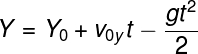Y – height

0 – initial height

v0y – initial vertical velocity

• ### Torricelli’s equation for the vertical launch

Finally, it is possible to relate height , velocity and gravity through the Torricelli equation The following equation is particularly useful when we don’t know what the rise time was , for example.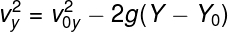vy – vertical speed

It is important to realize that the negative signs used in the vertical launch formulas relate to the fact that we are considering an upward vertical launch, which occurs against the direction of gravity. If we wanted to solve some exercise in which an object is thrown downwards, in favor of gravity, it would be enough to use the positive sign, since gravity would be in favor of the movement. Want to understand more about it? Access our text about vectors and learn how to use them.

## Free fall and vertical launch exercises

Question 1 – A 2 kg object falls from a certain height in relation to the ground, taking about 0.5 seconds to hit it. Disregarding the effect of dissipative forces, if a 4 kg object were dropped from the same height, the fall time of this body would be equal to:

Data : g = 10 m/s²

a) 1.0 s

b) 1.5 s

c) 0.5 s

d) 0.25 s

e) 0.75 s

Template: letter C. In free-fall movement, in which there is no effect of drag forces or friction with the air, objects of different masses reach the ground at equal intervals of time.

Question 2) A brick is thrown upward, in a vertical launch, with an initial speed of 10 m/s. Disregarding the effects of air resistance, determine the maximum height this brick is capable of reaching.

Data : g = 10 m/s²

a) 10 m

b) 5 m

c) 15 m

d) 7.5 m

e) 12 m

Template : letter b. To find the maximum height that the brick reaches, it is necessary to consider that the final speed of the ascent is equal to zero, in this case, we will use Torricelli’s equation. Watch: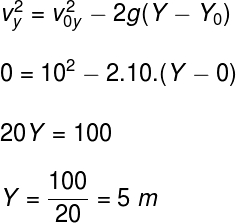To perform the above calculation, we adopted that the initial launch height was equal to zero, with this, the calculation indicated that, from the launch point, the brick rose 5 m in height, before starting the fall movement.

Question 3) When released in a vacuum, an object reaches the ground after a time of 1.5 s, in a place where gravity is equal to 10 m/s². Check the alternative that indicates the approximate height at which this object was dropped:

a) 15 m

b) 12 m

c) 5.25 m

d) 11.25 m

e) 17.5 m

Template : letter D. Using the free fall formula, we can determine the height of the object’s fall: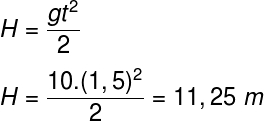Based on the data provided in the exercise, the calculation indicates that the height of the object was 11.25 m, so the correct alternative is the letter D.